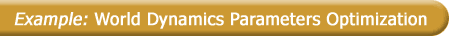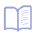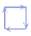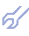The input data sampling was taken from fundamental work of Jay Forrester . There are presented: x1 = P - world population (in thousands); x2 = C - capital investment in industry (thousands of dollars); x3 = A - capital investment in agriculture (thousands of dollars); y1 = N - national resources increments (cond.units); y2 = F - pollution of environment (cond.units); y3 = I - quality of life index. The last three variables presents goal function vector. According to the SLP algorithm we are to point out the optimal values of its components. Let us take for example, as reference, the values, corresponding to year 1970: y1opt = -15920, y2opt = 5189.57; y3opt = 1.0. For first step we calculate deflections from optimal values for all the output variables: R1 = y1 - y1opt ; R2 = y2 - y2opt ; R3 = y3 - y3opt . Next step of the algorithm is to find the models of constrains by Combinatorial GMDH algorithm: R2 = 23383.770 - 7.497x1 + 31135.99x3 - 0.406y2 - 4031.958y3 ; RR = 0.0153; R3 = 13616.390 - 1.564x1 + 4.084x2 - 58020.660x3 - 8173.558y3 ; RR = 0.00083; R3 = 1.2641 - 0.000152x1 + 0.000432x2 - 7.101x3 - 0.0000019y1 - 0.0000967y2; RR = 0.0025; System of constrains equations is following R1 = 0; R2 = 0; R3 = 0. If we solve this system and substitute the meanings of functions, we get optimal values of input variables x1opt = 2931.408; x2opt = 1950.189; x3opt = 0.152 . If we shall keep these values of input variables next year (next point) we shall reach the reference values of output variables, pointed out above.